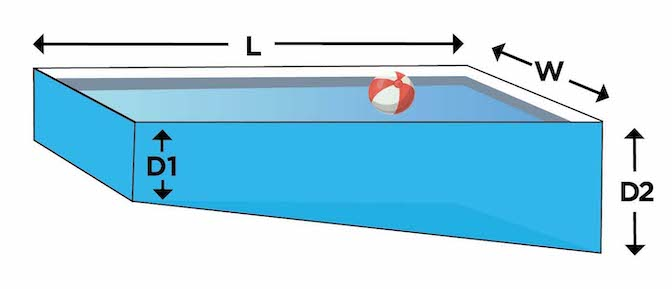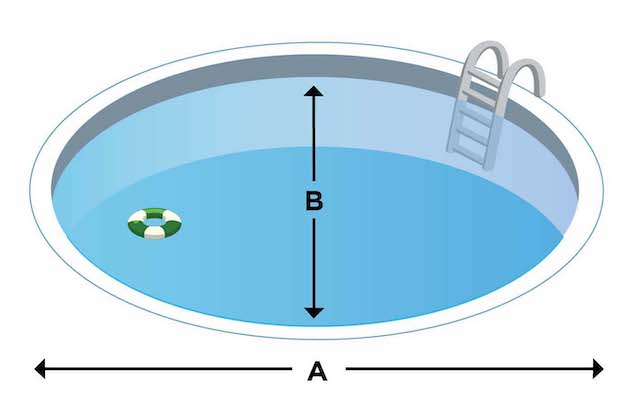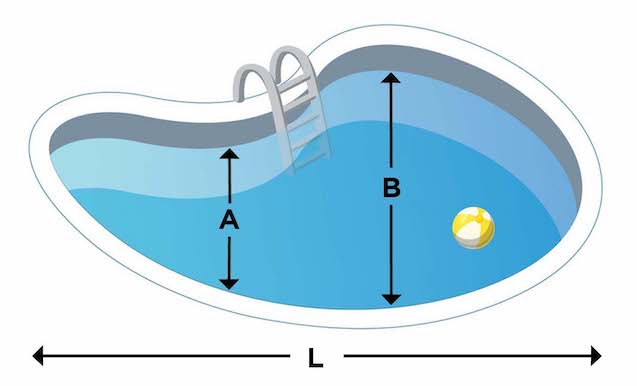There are many reasons you may need to know how many gallons of water your pool holds. This is useful for knowing how much of a certain chemical to add to your pool after you get a free 7-point water test at Pinch A Penny. It is also necessary for certain treatment programs, such as dealing with stains or algae.

Knowing the size of your pool is a must for any new equipment purchases as well. Whether it is a new pump or a pool heater, you will need to calculate the size of the equipment to see if it’s right for your pool. Fortunately, it’s not hard to calculate how many gallons are in your pool!#### Rectangular Pool

• Add the depth of the deep end and the depth of the shallow end, then divide by 2 to find the average depth.
• Multiply the length times the width, then multiply by the average depth from the previous step.
• Finally, multiply by 7.5.

For example, a pool that’s 30 feet long and 15 feet wide, with an average depth of 5 feet, would be 30 x 15 x 5 x 7.5 = 16,875 gallons.#### Oval/Round Pool

• For an oval pool, multiply the long diameter and short diameter (length across), then multiply by the average depth and 5.9.
• If your pool is a circle, use the previous calculation with the same diameter for both.#### Kidney-Shaped Pool

• For a kidney-shaped pool, you first need to calculate the surface area by adding the distance across from the two widest points (A + B) together.
• Multiply the surface area by the length and 0.45 to calculate the the total area.
• Multiply the total area by the average depth and 7.5 to get the gallonage of your pool.